# Reason - math word problems

1. DigitsShow that if x, y, z are 3 consecutive nonzero digits, zyx-xyz = 198, where zyx and xyz are three-digit numbers created from x, y, z.
2. Two rectanglesI cut out two rectangles with 54 cm², 90 cm². Their sides are expressed in whole centimeters. If I put these rectangles together I get a rectangle with an area of 144 cm2. What dimensions can this large rectangle have? Write all options. Explain your calc
3. Utopia IslandA probability of disease A on the island of Utopia is 40%. A probability of occurrence among the men of this island, which make up 60% of all the population (the rest are women), is 50%. What is the probability of occurrence of A disease among women on Ut
4. Glass with icecream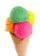We have 6 kinds of ice cream and 5 kinds of fruit. We put 3 cups of ice cream and 2 fruits into each glass. How many can unique decorated glasses be?
5. 40% volume40% volume with 104 uph (units per labor hour) 8 people working. What is the volume?
6. 3 cats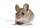3 cats eat 3 mice in 3 days. How many mice will eat 10 cats in 10 days?
7. Triangles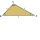Five sticks with a length of 2,3,4,5,6 cm. How many ways can you choose three sticks to form three sides of a triangle?
8. Right triangle eq2Find the lengths of the sides and the angles in the right triangle. Given area S = 210 and perimeter o = 70.
9. Gold inheritance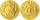The king divided the inheritance with his three sons in the ratio of 7: 6: 4. Two of them received 286,000 gold. How much each of the sons got.
10. Three friendsThree friends had balls in ratio 2: 7: 4 at the start of the game. Could they have the same number of balls at the end of the game? Write 0, if not, or write the minimum number of balls they had together.
11. RectanglesThe perimeter of a rectangle is 90 m. Divide it into three rectangles, the shorter side has all three rectangles the same, their longer sides are three consecutive natural numbers. What is the dimensions of each rectangle?If we read the book at a speed of 15 pages a day, we read it 3 days before we read it at a speed of 10 pages per day. If I read at 6 pages per day, how many days will I read the book?
13. Three students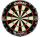Three students independently try to solve the problem. The first student will solve a similar problem with a probability of 0.6, the second student will solve at a probability of 0.55, and the third will solve at a probability 0.04. The problem is resolve
14. Clothes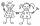Danka and Janka collect clothes. Danka had 9 more than Janka, so she gave her 7. Which now has more cloth and how many?
15. Bridge cards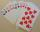How many bridge hands are possible containing 4 spades,6 diamonds, 1 club, and 2 hearts?
16. Four numbersThe first number is 50% second, the second number is 40% third, the third number is 20% of the fourth. The sum is 396. What are the numbers?
17. Buns and toastsTwo buns weigh 10 grams more than two toasts. One Bun and two Toast weigh a total of 110 grams. How many grams weigh three toasts? How many grams does a Bun weigh?
18. Repair pipe20 workers had to repair broken pipes in 30 days. After fourteen days, four laborers joined them. How long did the pipe repair work last?
19. SnacksThe school attends 344 pupils. Half of them take snacks. 13 pupils who took snacks did not attend school. How many snacks left?
20. GirlsThe boys and girls in the class formed without the rest of the fives, 2 girls and 3 boys. There are 6 girls missing to create mixed pairs (1 boy and 1 girl). How many girls are in the classroom?

Do you have an interesting mathematical word problem that you can't solve it? Submit math problem, and we can try to solve it.

We will send a solution to your e-mail address. Solved examples are also published here. Please enter the e-mail correctly and check whether you don't have a full mailbox.

Please do not submit problems from current active competitions such as Mathematical Olympiad, correspondence seminars etc...